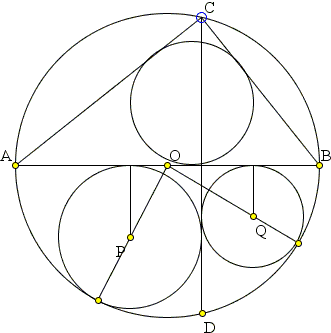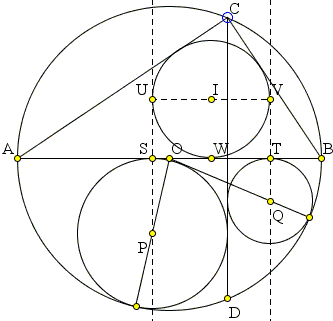## Arithmetic Mean Sangaku: What Is This About? A Mathematical Droodle

### This applet requires Sun's Java VM 2 which your browser may perceive as a popup. Which it is not. If you want to see the applet work, visit Sun's website at https://www.java.com/en/download/index.jsp, download and install Java VM and enjoy the applet.

 What if applet does not run?

Solution## SangakuThe applet is hopefully suggestive of the following sangaku [Bicycle, #39]:

 Points C and D lie on a circle with diameter AB, CD ⊥ AB. One circle is inscribed into ΔABC. Two more circles inscribed on the other side of AB in the curvilinear triangles formed by AB, CD and the given circle. Prove that the radius of the former is the average of the radii of the latter. Moreover, the lines through the centers of the latter circles are tangent to the former circle.

### This applet requires Sun's Java VM 2 which your browser may perceive as a popup. Which it is not. If you want to see the applet work, visit Sun's website at https://www.java.com/en/download/index.jsp, download and install Java VM and enjoy the applet.

 What if applet does not run?

We apply the Pythagorean theorem twice to the right triangles with hypotenuses joining the centers of the circles, as shown:Let the distance from center O of the circumcircle to CD be d, the other centers I, P, Q, and the radii of the circles be R, r, rL, rR. Then the Pythagorean theorem gives

 (r + d)2 + r2 = (R - r)2, for r = rR and (r - d)2 + r2 = (R - r)2, for r = rL,

From which we express rR and rL:

 (1) rR = -(R + d) + √2R(R + d) and rL = -(R - d) + √2R(R - d).

which shows that the arithmetic mean of the two equals

 (2) (rR + rL)/2 = -R + (√2R(R + d) + √2R(R - d) )/2.

One the other hand, we need to find the inradius r of the right ΔABC. For any right triangle with sides a, b and hypotenuse c, the inradius r can be found from

 r = (a + b - c)/2.

In our case, c = 2R, while a and b can again be found with the help of the Pythagorean theorem. It's not hard to see that one of them is 2R(R + d) whereas the other is 2R(R - d), which conforms with (2) and shows that the horizontal distance between P and Q is 2r. We may rewrite (1) as

 (1') rR = -(R + d) + b rL = -(R - d) + a.Thus ST = 2r, where S and T are the points of tangency of (P) and (Q) with AB. In particular,

 (3) r = (rR + rL)/2 = -R + (a + b)/2.

What remains to show is that the midpoint of ST is exactly the point of tangency W of the incircle (I) with AB, i.e. SW = TW = r.

Let's compute

 TW = BW - BT = (a - r) - (R - d - rR) = (a - r) - (2R - b) (by (1')) = (a+b) - r - 2R = (a+b) - 2R - r = 2r - r (by (3)) = r.

And we are done.

(This sangaku has a nice generalization.)

### References

1. H. Fukagawa, D. Pedoe, Japanese Temple Geometry Problems, The Charles Babbage Research Center, Winnipeg, 1989

Write to:

Charles Babbage Research Center
P.O. Box 272, St. Norbert Postal Station
Winnipeg, MB